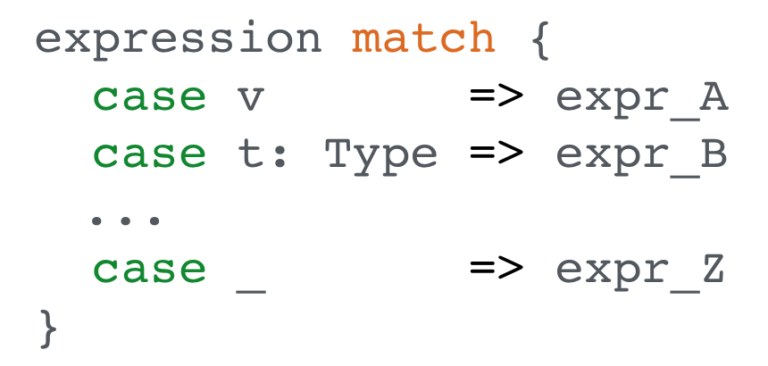# Scala: Pattern Matching

### Pattern matching in a programming language can make you a lot more productive and your code more readable. Find out how Scala helps you get there.

· Java Zone · Tutorial
Save
4.84K Viewsthere are a lot of cases when you need to make a decision in a program based on some condition or value. the first thought which comes to mind is an if else operator or something like switch in java. but scala offers a more powerful and elegant approach for the handling of such situations. let’s consider how pattern matching can help you.

here is a simple demonstration of pattern matching:

``````1 + 2 - 3 + 4 - 5 match {
case -1 => "minus one"
case 0  => "zero"
case 1  => "one"
case _  => "unknown"
}
//minus one``````

in the first line we define an algebraic expression, then we use a special keyword ``` match ``` . after that follows curly bracket. in lines 2, 3, 4 and 5 we put possible values. they represent cases which we expect to get as a result of expression evaluation. finally, the curly bracket is closed.

summed up here is the general syntax for pattern matching:we can ``` match ``` any expression, then in ``` case ``` sections we describe what we expect to get. if the expression matches some particular case, pattern matching stops and returns an expression which corresponds to the matched case. if there is no match, then a ``` matcherror ``` exception is thrown. that’s why, as a rule, in the last ``` case ``` line, developers put an underscore; it serves as a default expression.

## pattern matching examples

let’s examine several samples where pattern matching is used. the first example is the most simple. we are going to match expressions to constants.

``````val boo: boolean = 5 < 10

boo match {
case true => 1
case false => 0
}
//1``````

in the next example we place pattern matching in a function and use an underscore in case all previous cases are not matched.

``````def matchfunction(v: int) = v match {
case 1 => "one"
case 2 => "two"
case _ => "unknown number"
}

matchfunction(2) //two
matchfunction(5) //unknown number``````

now we can continue with more complex samples. let’s apply pattern matching to custom classes:

``````trait payment {
def pay(amount: double): unit
}

class cash extends payment {
def pay(amount: double): unit = println(s"pay with cash \$amount")
}
class creditcard extends payment {
def pay(amount: double): unit = println(s"pay with credit card \$amount")
def verify(): unit = println("verification...")
}

def processpayment(amount: double, method: payment) = method match {
case c: cash => c.pay(amount)
case cc: creditcard => cc.verify(); cc.pay(amount)
case _ => println("unknown payment method")
}

val paymenta = new cash
val paymentb = new creditcard

processpayment(10, paymenta)
//pay with cash 10.0

processpayment(50, paymentb)
//verification...
//pay with credit card 50.0``````

based on these three examples we can cover most parts of real life scenarios. furthermore, pattern matching can be nested. but, as is the case with if-else when level of nesting exceeds 2, the code can become hard to read.

## collection pattern matching

you can apply pattern matching to collections. it’s very convenient to deal with collection elements using pattern matching. here is basic example:

``````val commonlist = list(1, 2, 3, 4, 5)
val emptylist = nil
val oneelement = 'a' :: nil

def checklist[t](list: list[t]): string = list match {
case nil => "empty list"
case list :: nil => "one element"
case _ => "more than one element"
}

checklist(emptylist) //empty list
checklist(oneelement) //one element
checklist(commonlist) //more than one element``````

here is how you can use pattern matching in a recursive sum of integers.

``````val commonlist = list(1, 2, 3, 4, 5)
def sum(list: list[int]): int = {
def recursion(sum: int, list: list[int]): int = list match {
case nil => sum
case el :: tail => (recursion(sum + el, tail))
}
recursion(0, list)
}

sum(commonlist) //15``````

that’s why pattern matching so useful in scala. it’s very handy to operate with values without casting them using ``` instanceof ``` . also it’s much more readable than if-else chains.